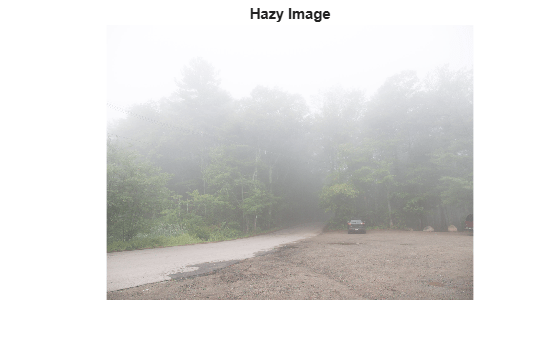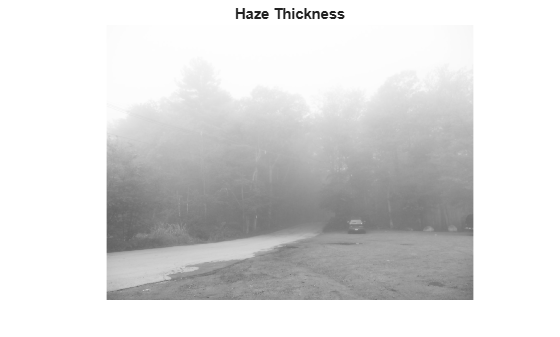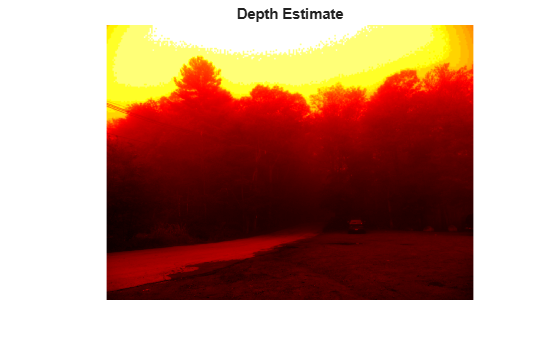Main Content

# imreducehaze

Reduce atmospheric haze

## Syntax

``[J,T,L] = imreducehaze(I)``
``[___] = imreducehaze(I,amount)``
``[___] = imreducehaze(___,Name,Value)``

## Description

example

````[J,T,L] = imreducehaze(I)` reduces atmospheric haze in color or grayscale image `I`. The function returns the dehazed image `J`, an estimate `T` of the haze thickness at each pixel, and the estimated atmospheric light `L`.```
````[___] = imreducehaze(I,amount)` additionally specifies the amount of haze to remove.```

example

````[___] = imreducehaze(___,Name,Value)` changes the behavior of the dehazing algorithm using name-value pairs.```

## Examples

collapse all

Read a hazy image into the workspace.

`A = imread('foggysf1.jpg');`

Reduce the haze and display the result next to the original image in a montage.

```B = imreducehaze(A); montage({A,B}) title("Hazy Image (Left) vs. Reduced Haze Image (Right)")```Read a hazy image into the workspace.

`A = imread('foggysf2.jpg');`

Reduce 90% of the haze using the `approxdcp` method.

`B = imreducehaze(A,0.9,'method','approxdcp');`

Display in a montage the original hazy image and the image with reduced haze.

`montage({A,B})`Read and display a hazy image.

```A = imread('foggyroad.jpg'); imshow(A) title('Hazy Image')```Reduce haze in the image using default parameter values. Return an estimate of the haze thickness.

`[~,T] = imreducehaze(A);`

Display the haze thickness measurement.

```imshow(T) title('Haze Thickness')```The haze thickness `T` provides a rough approximation of the depth `D` of the scene, defined up to an unknown multiplication factor. Add `eps` to avoid `log(0)`.

`D = -log(1-T+eps);`

Display the estimated depth in false color.

```imshow(D,[]) title('Depth Estimate') colormap hot```## Input Arguments

collapse all

Hazy image, specified as an RGB or grayscale image.

Data Types: `single` | `double` | `uint8` | `uint16`

Amount of haze to remove, specified as a number in the range [0,1]. When the value is `1`, `imreducehaze` reduces the maximum amount of haze. When the value is `0`, `imreducehaze` does not reduce haze and the input image is unchanged. Larger values can cause more severe color distortion.

Data Types: `single` | `double` | `int8` | `int16` | `int32` | `int64` | `uint8` | `uint16` | `uint32` | `uint64`

### Name-Value Pair Arguments

Specify optional comma-separated pairs of `Name,Value` arguments. `Name` is the argument name and `Value` is the corresponding value. `Name` must appear inside quotes. You can specify several name and value pair arguments in any order as `Name1,Value1,...,NameN,ValueN`.

Example: ```B = imreducehaze(A,0.9,'method','approxdcp');```

Technique used to reduce haze, specified as the comma-separated pair consisting of `'Method'` and one of these values:

• `'simpledcp'` — Simple dark channel prior method . This technique uses a per-pixel dark channel to estimate haze and quadtree decomposition to estimate the atmospheric light.

• `'approxdcp'` — Approximate dark channel prior method . This technique uses both per-pixel and spatial blocks when computing the dark channel and does not use quadtree decomposition.

For more information, see Algorithms.

Data Types: `char` | `string`

Maximum value to be treated as haze, specified as the comma-separated pair consisting of `'AtmosphericLight'` and a 1-by-3 numeric vector for RGB images or a numeric scalar for grayscale images. Values must be in the range [0, 1]. Atmospheric light values greater than 0.5 tend to give better results.

If you do not specify `AtmosphericLight`, then the `imreduzehaze` function estimates a value depending on the value of `'method'`.

Data Types: `double`

Contrast enhancement technique, specified as the comma-separated pair consisting of `'ContrastEnhancement'` and `'global'`, `'boost'`, or `'none'`.

Data Types: `char` | `string`

Amount of per-pixel gain to apply as postprocessing, specified as the comma-separated pair consisting of `'BoostAmount'` and a number in the range [0, 1]. This argument is only supported if `ContrastEnhancement` is specified as `'boost'`.

Data Types: `double`

## Output Arguments

collapse all

Dehazed image, returned as numeric array of the same size as the input hazy image `I`.

Haze thickness estimated at each pixel, returned as a numeric array.

Estimated atmospheric light, returned as a numeric array. `L` represents the value of the brightest nonspecular haze.

## Algorithms

The model to describe a hazy image `I` is

`I(x) = J(x)T(x) + L(1-T(x))`
`I` is the observed intensity, `J` is the scene radiance, `L` is atmospheric light, and `T` is a transmission map describing the portion of light that reaches the camera.

Dehazing algorithms recover the scene radiance (dehazed image) `J` from an estimation of the transmission map and atmospheric light according to:

`J(x) = (I(x)-A)/(max(t(x),t0)) + A`

`imreducehaze` uses two different dehazing algorithms, `simpledcp` and `approxdcp`. These methods both rely on a dark channel prior, which is based on the observation that unhazy images of outdoor scenes usually contain some pixels that have low signal in one or more color channels. The methods differ in how they estimate the dark channel prior and atmospheric light.

The dehazing algorithms in `imreducehaze` follow five steps:

1. Estimate the atmospheric light `L` using a dark channel prior.

2. Estimate the transmission map `T`.

3. Refine the estimated transmission map.

4. Restore the image.

5. Perform optional contrast enhancement.

 He, Kaiming. "Single Image Haze Removal Using Dark Channel Prior." Thesis, The Chinese University of Hong Kong. 2011.

 Dubok, et al. "Single Image Dehazing with Image Entropy and Information Fidelity." ICIP. 2014, pp. 4037–4041.

Download ebook